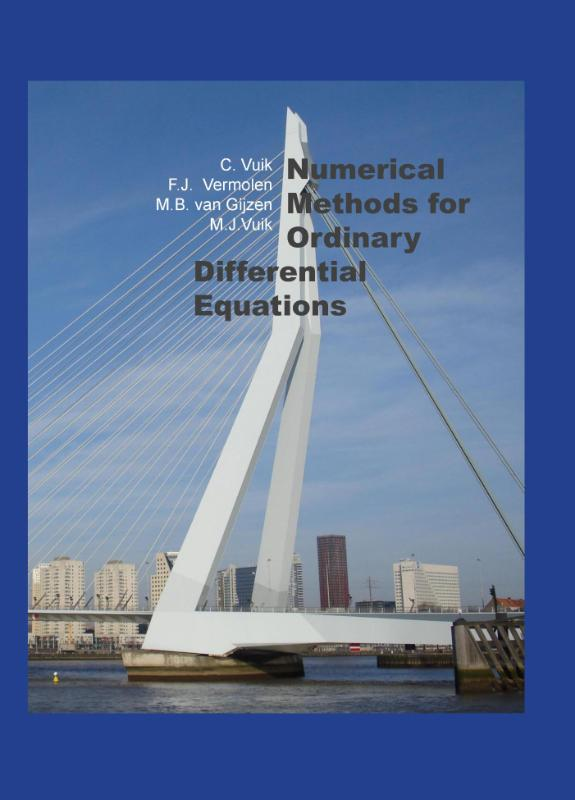Wij werken op volle kracht, er is geen vertraging in productie en levering door het Coronavirus. Meer informatie via deze link.

### Niet leverbaar

×ePUB ebook

niet beschikbaar

PDF ebook

niet beschikbaar

# Numerical methods for ordinary differential equations

### M.J. Vuik • Boek • paperback

• Samenvatting
Numerical Methods for Ordinary Differential Equations In this book we discuss several numerical methods for solving ordinary differential equations. We emphasize the aspects that play an important role in practical problems. We confine ourselves to ordinary differential equations with the exception of the last chapter in which we discuss the heat equation, a parabolic partial differential equation. The techniques discussed in the introductory chapters, for instance interpolation, numerical quadrature and the solution to nonlinear equations, may also be used outside the context of differential equations. They have been included to make the book self-contained as far as the numerical aspects are concerned. Contents: Preface - 1. Introduction - 2. Interpolation - 3. Numerical differentiation - 4.Nonlinear equations - 5. Numerical integration - 6. Numerical time integration of initial-value problems - 7. The finite-difference method for boundary-value problems - 8. The instationary heat equation- Literature - Index
• Productinformatie
Binding : Paperback
Distributievorm : Boek (print, druk)
Formaat : 169mm x 240mm
Aantal pagina's : 123
ISBN : 9789065623737
Datum publicatie : 02-2015
• Inhoudsopgave
niet beschikbaar
• Reviews (0 uit 0 reviews)

# € 16,40niet beschikbaarniet beschikbaar

Niet leverbaar
Veilig betalen14 dagen bedenktermijn
Delen

niet beschikbaar

×
Contact